X

Select the account you'd like to open

# What is the MACD?

The moving average convergence divergence (MACD) is a simple, yet effective indicator. Learn how to interpret and use the MACD for technical trading.

It can provide a visual snapshot to help analyse trends, allowing traders to scan charts rapidly. That makes it an invaluable technical analysis tool, especially in today’s ‘global village’ with myriad financial instruments available to traders at the click of a mouse.

The MACD is a trend-following momentum indicator/oscillator, developed by Gerald Appel in the late-1970s. It is used to determine the strength and momentum of a trend, and is calculated on price data which is plotted as a time series.

The idea behind the MACD is simple. The degree/magnitude of separation between a shorter- and a longer-term moving average (MA) denotes the strength of a trend. It also indicates the momentum of that trend. The underlying logic is that a shorter-term MA reflects current price action; whereas a longer-term MA reflects earlier price action, in addition to the current price action. If there is good separation between these two MAs, it means that current price action is moving away from earlier price action. This indicates that the market is trending either up or down.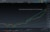From CMC Market’s Next Generation Platform: Exponential Moving Average Illustration of a Trend

A time series is simply a set of data plotted over time. An example of a time series is temperature data (high, low, average) plotted daily. Financial Instruments traded in the market are plotted as price data that is continuously recorded. Prices may be plotted for a certain number of transactions (ticks). They may also be plotted in time periods, of usually one second and upwards. They are plotted on a chart as the open-high-low-close for that period; either as an open-high-low-close (OHLC bar), or as a candlestick.

An MA is an average of time series data calculated over a certain number of time periods. As we progress through time, the latest period factors into the calculation, and the earliest period from the previous calculation drops off. Another thing to note is that a faster MA is defined as one that is calculated over a smaller number of periods. Conversely, a slower MA is one calculated over a larger number of periods. Calculated MAs are consequently plotted on the charts and are usually overlaid on top of the price action.

MAs come in various flavours, but some may be more relevant in understanding the MACD. A simple moving average (SMA) is an average of data plotted over a certain number of periods. A weighted moving average (WMA) is a moving average with greater emphasis (or weight) being placed on certain (or few) periods.

We also have the exponential moving average (EMA). This is where more recent periods have a higher weight in the calculation, compared to earlier periods. This is done using a weighting multiplier calculated as 2/(time period + 1). Therefore for a nine-period time series it is 2/(9 + 1) which is 0.2 or 20%. Given that the logic behind the MACD is to compare recent versus earlier price action, an EMA is more applicable and therefore used in its calculation.

## The MACD indicator explained

Interpreting MACD on charts has been the focus of traders seeking to identify a market’s trend and momentum since the MACD indicator’s inception. It is easy to use and can, at a glance, give a myriad of information to the astute trader.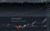From CMC Market’s Next Generation Platform: The MACD Indicator

## How to use the MACD indicator

Prices of all financial instruments do not move in a straight line, but rather in a jagged up and down fashion with a series of sharp peaks and troughs. Examples of financial instruments are the foreign exchange market, equities, indices, commodities, exchange traded funds (ETFs), debt instruments (bonds), futures and options. Learn more about the similarities and differences between futures and options. These fluctuations and oscillations are filtered out by low-pass filters. MAs present a smoother profile, which allows for ease of analysis. The MACD formula seeks to harness the benefits of two low-pass filters: a fast EMA and a slow EMA. The standard or “box” setting for the fast EMA is 12 periods, for example an EMA calculated over 12 periods. The standard or “box” setting for the slow EMA is 26 periods, for example an EMA calculated over 26 periods.

The MACD indicator consists of signal lines and a histogram. The histogram displays the difference between the MACD line (more commonly referred to simply as MACD) and the signal line plotted as a bar chart over time.

The MACD can be used in several ways by traders. At a very basic level it is used to generate buy and/or sell signals using crossovers. When the MACD crosses the signal line from under it and goes over, a buy signal is generated. Such a crossover is often known as the “golden cross”. Conversely, when the MACD crosses the signal line from above and goes under it, this generates a sell signal, and is often known as the “death cross”.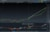From CMC Market’s Next Generation Platform: The MACD Golden Cross

The MACD histograms oscillate above and below a “zero line”, where the MACD and the signal line intersect. The shape of the histogram is important, for example a rising histogram profile indicates an uptrend. The shape of the histogram with respect to the zero line also has a bearing on the trend as a strong downtrend would be indicated by a falling profile below the zero line.

The MACD is one of the earliest indicators developed and yet it still remains effective in today’s modern markets. For instance, it can be used as a leading indicator to predict potential market direction in conjunction with price action.

The way to do this is by using the concepts of convergence and divergence. The MACD is said to be convergent with price action if both prices and the MACD are moving in the same direction. In other words, if prices are making higher highs (in an uptrend) with the MACD makes correspondingly higher highs then we have convergence. Convergence of the MACD with price action confirms the strength, direction, and momentum of a trend.
If the MACD moves in the opposite direction, making lower highs as price is making higher highs, then we have negative divergence. Divergence with price action indicates the potential weakening or possible reversal of a trend.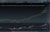From CMC Market’s Next Generation Platform: MACD Convergence

## Calculating the MACD

The mathematics behind MACD is relatively simple and powerful when used effectively. The MACD is calculated by subtracting the slow EMA from the fast EMA. In the example below, the fast EMA (12) has a value of 6,151.99 and the slow EMA (26) has a value of 5554.52. The resultant MACD value is 597.16.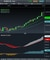From CMC Market’s Next Generation Platform: Calculating MACD

## What is the MACD Signal line?

The MACD signal line is the second component of the MACD indicator. It is an EMA of the MACD over a certain number of periods. The standard or “box” setting for this is nine, in other words an EMA of the MACD calculated over nine periods. It is used to generate buy and sell signals as the MACD line crosses.

As the MACD and MACD signal line are derived from two EMAs, their value will be dependent on the underlying security. As such, it is not possible to compare these values for a group of currencies or across markets such as between the US SPX 500 and an exchange traded fund.

## The benefits of MACD

The MACD is a relatively simple indicator, easy to comprehend, appeals to intuitive logic and therefore resonates well with most traders. It can be a powerful tool if used effectively, especially for assessing the strength and momentum of trends, and consequently to predict their continuance and potential reversal.

Live account

Access our full range of markets, trading tools and features.

Open a live account

Demo account

Try CFD trading with virtual funds in a risk-free environment.

Open a demo account